Wednesday, May 4, 2011

Jomari's Post for May 4, 2011

Today we learned about perpendicular bisectors.
These are lines that bisect straight lines at 90°.
Here's how to make one:

We made these bisectors for several chords.In a circle, we don't need to make crossed arcs on one side of the chord because of the center.
After wards, we answered a problem involving bisectors. It was similar to this:
A wheel is stuck in some water. The radius is 22cm and the water takes up 34cm lengthwise of the wheel. How deep is the wheel?
This is what we have to work with: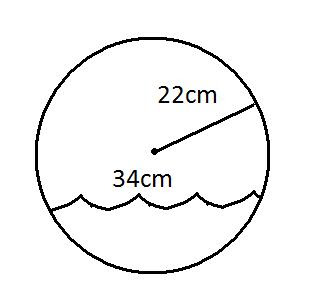This question was difficult because of the way it was drawn, but he then realized we could do this: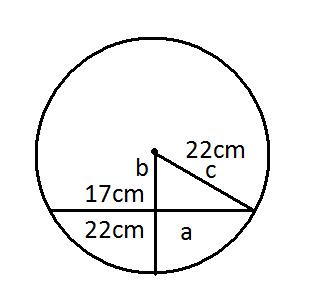WE COULD USE THE PYTHAGORAS THEOREM TO FIND B
a
²+b²=c²
17
²+b²=22²
289+b²=484
b²=484-289
b²=195
√ b²=√ 195²
b=13.96

22cm-13.96cm=8.04cm
THE WHEEL IS 8.04cm deep into the water

Tuesday, May 3, 2011

Scribepost May 3, 2011

Today in Math, we continued on circle geometry.

Basically, Backé taught us more on how to figure out the the angle of an inscribed angle by looking at its central angle. He also gave us words and diagrams that we need to know.

First, he showed us something we should know already. Here are kinds of triangles according to sides.....Equilateral Triangles are triangles with 3 equal sides, Isosceles Triangles have 2 equal sides, while Scalene Triangles have no sides equal.

....and here are other triangles according to their angles.Acute Triangles have 3 acute angles, Right triangles have 1 right angle and 2 acute angles, Obtuse Triangles have 1 obtuse angle and 2 acute angles.

He mentioned the word "Theta" which means an unknown angle.Then he gave us this table....It simply means that
• an angle that has an angle between 0 and 90 degrees is called an acute angle.
• an angle that has an angle of 90 degrees is called a right angle.
• an angle that has an angle between 90 and 180 degrees is called an obtuse angle.
• an angle that has an angle of 180 is called straight angle.
• angles that has an angle between 180 and 360 are called reflex angles.
wait there's more...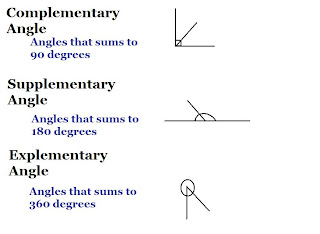He also showed us facts about the total angle of polygons.
- sum of all angles of a triangle = 180 degrees
- sum of all angles of a quadrilateral = 360 degrees

------------------------------------------------------------------------------------------------
That is about it. Comment if I did anything wrong or if I missed stuff.

***Bring a PROTRACTOR with you because we'll be needing it in this chapter***

Monday, May 2, 2011

Hannah's post for May 2, 2011

So today we started on chapter 10, which is all about angles in a circle.

For starters, some words that we need to know are:

1. chord: a line segment with both endpoints on a circle

2. central angle: an angle formed by two radii of a circle

3. inscribed angle: an angle formed by two chords that share a common point

4. arc (of a circle): a portion of the circumference

We drew four different diagrams in class. All of which involving a circle.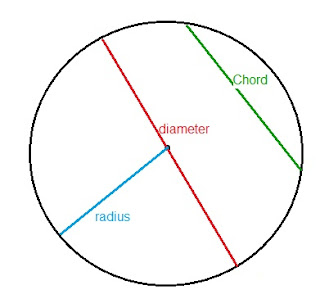They're subtended by the same arc. In other words, they share a common end point.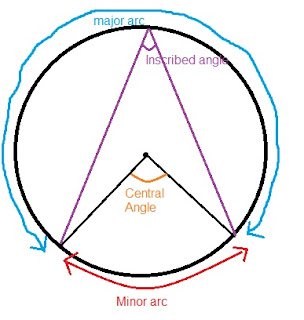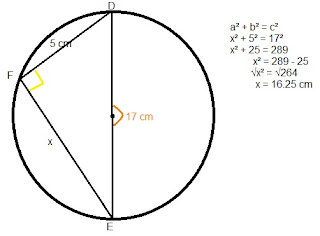Homework for tonight is..
• read pg. 382 Key Ideas
• CYU #1
• Practice and apply
• Extend 18, 19, 20
• Extra Practice
• Homework book

Monday, April 25, 2011

Jennifer's Post for April 25th, 2011

Hello everyone!

We started on a new chapter on Inequalities so far in math class, Mr.Backe started us off with an equation plus we learned how to do a solution set for x.The one with the bracket in the middle is the Solution set. Pretty much it's like a word answer but in math form. We also did some other questions similar in class as well. Here's some photos of some of the questions we did in class and how I solve it.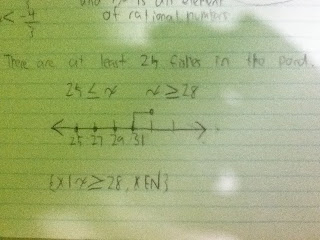Thats All for now!

Monday, April 18, 2011

Jomari's Post for April 18, 2011

Today we corrected our tests. In the last 5 minutes of class we went to
http://www.math.com/school/subject2/lessons/S2U1L4GL.html
Here's most of itAlso remember that we have to hand in our stash-it soon.

Tuesday, April 5, 2011

Jem's Post for April 5, 2011

Hi guys, if i haven't welcomed you guys yet .. welcome back! Right when class started Mr.Backe reminded us about everything due on Friday. Then everyone was busy.

1. Chapter 7 ; Self Assessment
Problems of the Week # 1, 2, 3
Eg ; For #3 we had a little lesson on the Fibonacci sequence like Emmanuel explained "The Fibonacci sequence, is a pattern where a number plus the number before are added together to get the next number 1,1,2,3,5,8 ... etc"
Polynomial Puzzle Sheet
2. Chapter 8; Foldable ( Attach your chapter 8 Self Assessment to Show You Know)
Problem of the Week # 3, 4

For today's class that's all he went through, unless of course you need to ask him questions. Then the rest of the class was working period, GET YOUR WORK DONE GUYS!

For more information visit Mr.Backe : Room 5 (morning, lunch, and afterschool)

Wednesday, March 23, 2011

Jem's Blog for March 22, 2011

Hello 9-05 and hello world,

So yesterday for math class our test was posponed, due to lack of understanding questions and how to solve them. Anyways, to help us understand the "hard" questions Mr.Backe went over questions with us, and made sure that we understand what the questions mean, and how to solve them.. Lets begin :
1. 4 - 6 = 7 +2
___ ______ ( Solve what you can and need to first )
x + 2 2( x - 4 )
- 2 9
___ = ______ ( From here you can now cross multiply )
x + 2 2x - 8

-2 ( 2x + 8 ) = 9x + 18 ( Now you solve what you can and need to again )

-4x - 16 = 9x + 18 ( Then you gather all the like terms )

-16 - 18 = 9x + 4x

- 2 = 13 x
____ ____ ( Last step is to isolate the variable )
13 13

-2
_____ = x
13

1. B scored 78% on its test. B had 11 correct answers. What was the test out of?
We always start with what we know. We know that he scored 78% and got 11 right, we can make the equation and just solve for x.

78 = 11
___ ____
100 x

78x = 1100
____ ____
78 78
x = you'll get an ugly number so just round the number.

Then we did other questions, that are similar to those. So just make sure you understand if not GO TO MATH HELP PLEASE. Good luck guys!